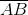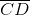## EX 14.1 QUESTION 1.

Draw a circle of radius 3.2 cm.

Solution:Step I: Open the compass up to the given radius of 3.2 cm.

Step II: Mark a point O as a centre.

Step III: Place the needle of the compass at the centre O.

Step IV: Holding the top of the compass and turn the compass slowly to draw the circle.

## EX 14.1 QUESTION 2.

With the same centre O, draw two circles of radii 4 cm and 2.5 cm.

Solution:Step I: Mark a point O as a centre.

Step II: Open the compass up to 4 cm

Step III: Place the needle of the compass at the centre O.

Step II: Draw a circle keeping the needle fixed at O.

Step IV: Holding the top of the compass and turn the compass slowly to draw the circle.

Step V: Again Open the compass up to 2.5 cm.

Step VI: Take the same centre O and draw another circle.

## EX 14.1 QUESTION 3.

Draw a circle and any two of its diameters. If you join the ends of these diameters, what is the figure obtained? What figure is obtained if the diameters are perpendicular to each other? How do you check your answer?

Solution:

(i) Draw a circle with centre 0 with a suitable radius.

(ii) AB and CD are any two diameters.

(iii) On joining the end points of the diameters, we get a quadrilateral ACBD.

(iv) We note that OA = OB = OC = OD [Same radius]and AC = DB, AD = BC

∠A = ∠C = ∠B = ∠D = 90°

Thus ACBD is a rectangle.

Again if the diameters are perpendicular to each other then on measuring, we get

AC = DB = AD = BC

Thus, ACBD is a square

## EX 14.1 QUESTION 4.

Draw any circle and mark points A, B and C such that

(a) A is on the circle.

(b) B is in the interior of the circle.

(c) C is in the exterior of the circle.

Solution:## EX 14.1 QUESTION 5.

Let A, B be the centres of two circles of equal radii; draw them so that each one of them passes through the centre of the other. Let them intersect at C and D. Examine whetherandare at right angles.

Solution:Draw two circles having with the same radius which are passing through the centre of the other circle. They intersect each other at point C and D. Join points AB and CD. We see thatandintersect at a right angle.

error: Content is protected !!